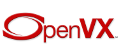The OpenVX Specification  r28647
Vision Functions

## Detailed Description

These are the base vision functions supported in OpenVX 1.0.

These functions were chosen as a subset of a larger pool of possible functions that fall under the following criteria:

• Applicable to Acceleration Hardware
• Very Common Usage
• Encumbrance Free

## Modules

Absolute Difference
Computes the absolute difference between two images.

Accumulate
Accumulates an input image into output image.

Accumulate Squared
Accumulates a squared value from an input image to an output image.

Accumulate Weighted
Accumulates a weighted value from an input image to an output image.

Arithmetic Subtraction
Performs subtraction between two images.

Bitwise AND
Performs a bitwise AND operation between two VX_DF_IMAGE_U8 images.

Bitwise EXCLUSIVE OR
Performs a bitwise EXCLUSIVE OR (XOR) operation between two VX_DF_IMAGE_U8 images.

Bitwise INCLUSIVE OR
Performs a bitwise INCLUSIVE OR operation between two VX_DF_IMAGE_U8 images.

Bitwise NOT
Performs a bitwise NOT operation on a VX_DF_IMAGE_U8 input image.

Box Filter
Computes a Box filter over a window of the input image.

Canny Edge Detector
Provides a Canny edge detector kernel.

Channel Combine
Implements the Channel Combine Kernel.

Channel Extract
Implements the Channel Extraction Kernel.

Color Convert
Implementes the Color Conversion Kernel.

Convert Bit depth
Converts image bit depth.

Custom Convolution
Convolves the input with the client supplied convolution matrix.

Dilate Image
Implements Dilation, which grows the white space in a VX_DF_IMAGE_U8 Boolean image.

Equalize Histogram
Equalizes the histogram of a grayscale image.

Erode Image
Implements Erosion, which shrinks the white space in a VX_DF_IMAGE_U8 Boolean image.

Fast Corners
Computes the corners in an image using a method based upon FAST9 algorithm suggested in  and with some updates from  with modifications described below.

Gaussian Filter
Computes a Gaussian filter over a window of the input image.

Harris Corners
Computes the Harris Corners of an image.

Histogram
Generates a distribution from an image.

Gaussian Image Pyramid
Computes a Gaussian Image Pyramid from an input image.

Integral Image
Computes the integral image of the input.

Magnitude
Implements the Gradient Magnitude Computation Kernel.

Mean and Standard Deviation
Computes the mean pixel value and the standard deviation of the pixels in the input image (which has a dimension width and height).

Median Filter
Computes a median pixel value over a window of the input image.

Min, Max Location
Finds the minimum and maximum values in an image and a location for each.

Optical Flow Pyramid (LK)
Computes the optical flow using the Lucas-Kanade method between two pyramid images.

Phase
Implements the Gradient Phase Computation Kernel.

Pixel-wise Multiplication
Performs element-wise multiplication between two images and a scalar value.

Remap
Maps output pixels in an image from input pixels in an image.

Scale Image
Implements the Image Resizing Kernel.

Sobel 3x3
Implements the Sobel Image Filter Kernel.

TableLookup
Implements the Table Lookup Image Kernel.

Thresholding
Thresholds an input image and produces an output Boolean image.

Warp Affine
Performs an affine transform on an image.

Warp Perspective
Performs a perspective transform on an image.